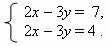# Systems of two simultaneous linear equations in two unknowns

Systems of two simultaneous linear equations in two unknowns.
Basic methods of solution. Substitution. Addition or subtraction
of equations. The second order determinants. Cramer's rule.
Investigation of solutions.

Systems of two simultaneous linear equations in two unknowns have the shape: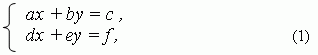where a, b, c, d, e, f – numerical coefficients; x, y – unknowns.

Solution of these simultaneous equations can be found by two basic methods:

Substitution. 1). From one equation we express one of unknowns, for example x , by coefficients and another unknown y :

x = ( c – by ) / a , (2)

2). Substitute in the second equation instead of x :

d ( c – by ) / a + ey = f .

3). Now, solving the last equation, find y :

y = ( af – cd ) / ( ae – bd ).

4). Substitute this value for y in the expression (2) instead of y :

x = ( ce – bf ) / ( ae – bd ) .

E x a m p l e .  Solve the system of simultaneous equations: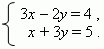From the first equation express x by coefficients and y :

x = ( 2 y + 4 ) / 3 .

Substitute this expression into the second equation and find y :

( 2 y + 4 ) / 3 + 3 y = 5 , hence y = 1 .

Now find x , substituting the found value instead of y into

expression for x : x = ( 2 · 1 + 4 ) / 3 , from here x = 2 .

Addition or subtraction. This method consists in the following.

1).  Multiply both sides of the first equation of the system (1) by ( d ) and both sides

of the second equation by a and add them: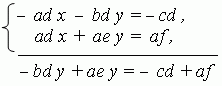From here we receive: y = ( af – cd ) / ( ae – bd ) .

2).  Substitute the found value of y into any equation of the original system (1) :

ax + b( af – cd ) / ( ae – bd ) = c .

3).  Find another unknown x : x = ( ce – bf ) / ( ae – bd ) .

E x a m p l e . Solve the system of simultaneous equations: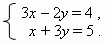by the second way  ( addition or subtraction ).

Multiply the first equation by  –1,  the second by 3 and add them: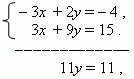From here y = 1 . Substitute this value into the second equation

( is it possible to substitute this into the first equation ? ): 3 x + 9 = 15, hence, x = 2 .

The second order determinants. We saw, that formulas for solution of the system of two simultaneous linear equations in two unknowns have the shape:

x = ( ce – bf ) / ( ae – bd ) ,

(3)

y = ( af – cd ) / ( ae – bd ) .

These formulas can be remembered very easily, if to introduce for their numerators and denominators the next symbol: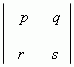,  which will be used to mean an expression:  ps – qr .

This expression is received by crosswise multiplication of numbers p , q , r , s :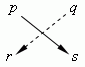and the following subtraction of one product from another: ps – qr. The sign  “+” is taken for a product of numbers, located on the diagonal, going from the left upper number to the right lower number. The sign “ “ for another diagonal, going from the right upper number to the left lower number. For example,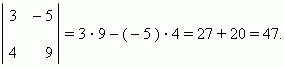The expression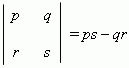is called the second order determinant .

Cramer’s rule. Using the determinants, the formulas (3) can be written as: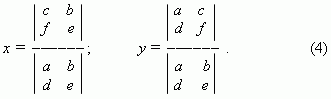Formulas ( 4 ) are called Cramer’s rule for solution of the system of  two simultaneous linear equations in two unknowns.

E x a m p l e .  Solve the system of simultaneous equations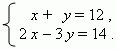using Cramer’s rule.

S o l u t i o n . Here a = 1, b = 1, c = 12, d = 2, e = 3, f = 14 .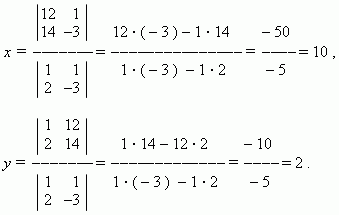Investigation of solutions of a system of two simultaneous linear equations in two unknowns shows, that depending on coefficients three different cases are possible:

1) coefficients at unknowns in equations are disproportionate : a : d b : e ,

in this case the system of  simultaneous linear equations has a single solution ,

presented by formulas (4) ;

2) all coefficients of equations are proportional : a: d = b: e = c: f , in this case

the system of simultaneous linear equations has an infinite set of solutions ,

because we have actually one equation instead of two.

E x a m p l e . In the system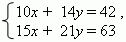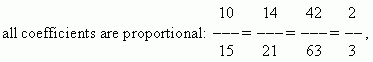and this system has an infinite set of solutions. ( Why? )

Dividing the first equation by 2 and the second - by 3,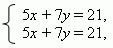that is one equation in two unknowns, which has an infinite set of solutions.

3) coefficients at unknowns are proportional, but disproportionate to free terms:

a : d = b : e c : f , in this case the system of simultaneous linear equations has

no solutions , because we have here contradictory equations.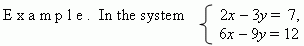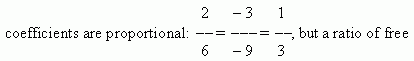terms is 7/12, not equal to 1/3. Why has not this system solution?

An answer is very easy. If to divide the second equation by 3,